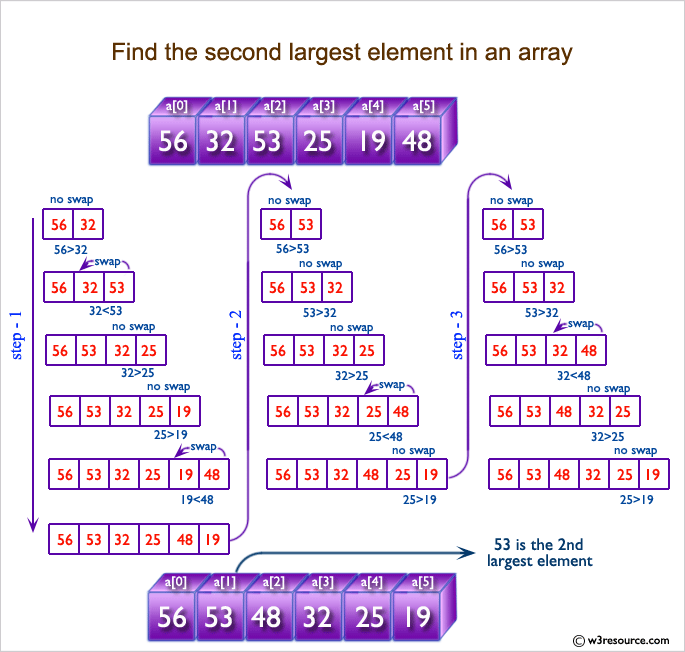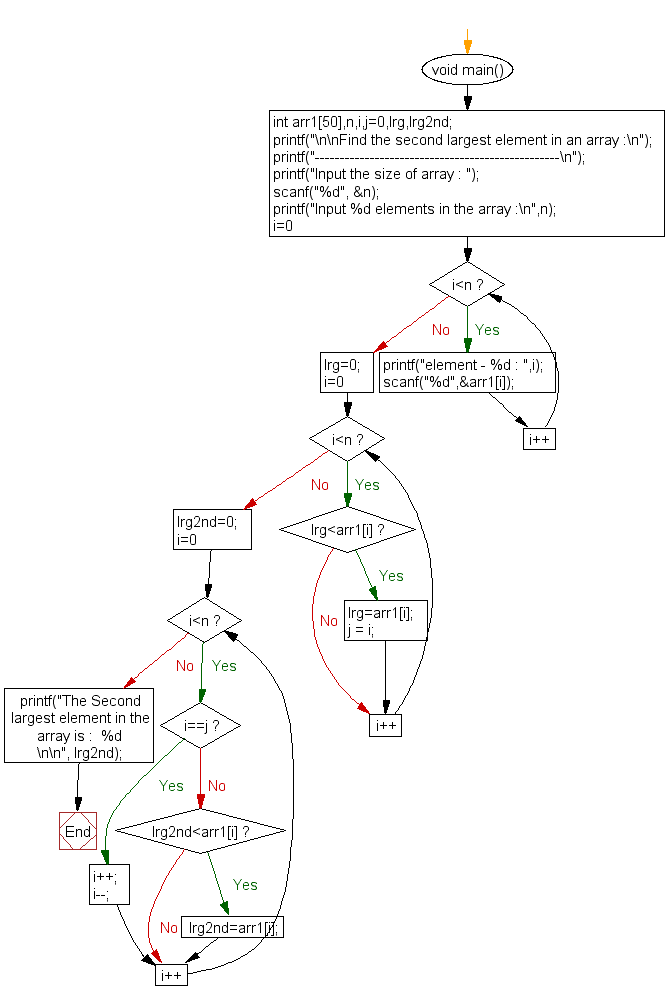﻿ C exercises: Find the second largest element in an array - w3resource# C Exercises: Find the second largest element in an array

## C Array: Exercise-16 with Solution

Write a program in C to find the second largest element in an array.

Pictorial Presentation:Sample Solution:

C Code:

``````#include <stdio.h>

void main(){
int arr1,n,i,j=0,lrg,lrg2nd;

printf("\n\nFind the second largest element in an array :\n");
printf("-------------------------------------------------\n");

printf("Input the size of array : ");
scanf("%d", &n);
/* Stored values into the array*/
printf("Input %d elements in the array :\n",n);
for(i=0;i<n;i++)
{
printf("element - %d : ",i);
scanf("%d",&arr1[i]);
}
/* find location of the largest element in the array */
//   lrg=arr1;
lrg=0;
for(i=0;i<n;i++)
{
if(lrg<arr1[i])
{
lrg=arr1[i];
j = i;
}
}

/* ignore the largest element and find the 2nd largest element in the array */
lrg2nd=0;
for(i=0;i<n;i++)
{
if(i==j)
{
i++;  /* ignoring the largest element */
i--;
}
else
{
if(lrg2nd<arr1[i])
{
lrg2nd=arr1[i];
}
}
}

printf("The Second largest element in the array is :  %d \n\n", lrg2nd);
}
```
```

Sample Output:

```Find the second largest element in an array :
-------------------------------------------------
Input the size of array : 5
Input 5 elements in the array :
element - 0 : 2
element - 1 : 9
element - 2 : 1
element - 3 : 4
element - 4 : 6
The Second largest element in the array is : 6
```

Flowchart:C Programming Code Editor:

Improve this sample solution and post your code through Disqus.

What is the difficulty level of this exercise?

﻿